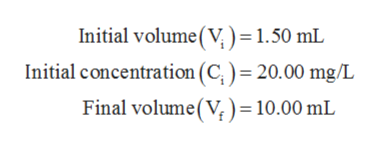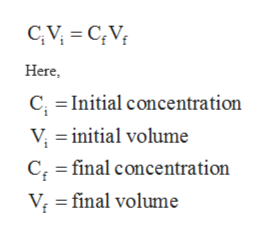Calculate the final concentration of a solution prepared by diluting 1.50 mL of a 20.00 mg/L solution of Fe 2+ ions to a final volume of 10.00 mL.

Question

Calculate the final concentration of a solution prepared by diluting 1.50 mL of a 20.00 mg/L solution of Fe 2+ ions to a final volume of 10.00 mL.

Step 1

The values are given as follows:help_outlineImage TranscriptioncloseInitial volume(V = 1.50 mL Initial concentration (C)= 20.00 mg/L Final volume(V) = 10.00 mL fullscreen
Step 2

The gram equivalent weight of a solute per liter of solution is considered as normality of the solution.

Step 3

The dilution equation can be represented in order to calc...help_outlineImage TranscriptioncloseCAV CV Here C, Initial concentration V initial volume C final concentration V final volume fullscreen

Want to see the full answer?

See Solution

Want to see this answer and more?

Our solutions are written by experts, many with advanced degrees, and available 24/7

See Solution
Tagged in

Chemistry